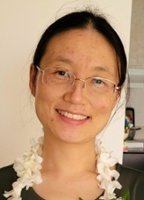# Rong Zou PH.D.

##### College of Natural and Computational Sciences - Department of Mathematics

Lecturer of Mathematics

Education:

• Ph.D. in Applied Mathematics, Kyushu University, Japan
• B.E. in Environmental Engineering, Huazhong University of Science and Technology, China

Courses Taught:

• MATH 1105: Intermediate Algebra
• MATH 1123: Statistics
• MATH 1140: Pre-Calculus II

Professional Interests:

Fluid dynamics problems, such as waves and instabilities in neutral fluids and in electrically conducting fluids, including Alfvén wave, Gravity wave, Magnetorotational instability, Tayler instability, Kelvin-Helmholtz instability, and so on. Mathematical modeling, Data analysis and Optimization problems, such as fitting data with the discrete choice models, trajectory optimization problems.

Teaching Interests/Research:

I am interested in teaching applied mathematics courses, including Probability theory, Statistics, Differential equations, Algebra, and mentoring undergraduate students and graduate students who have curiosity and enthusiasm to explore and solve physical and real-world problems, such as fluid dynamics, mathematical modeling, data analysis, optimization problems by using both theorical and numerical mathematics methods.

Personal Interests/Biography:

I have been living, studying, and working in China, Japan and Hawaii. I like to travel and to learn about different cultures, knowledge, and cuisines.Lecturer
PH.D.

Send email
(808) 543-8074
500 Ala Moana Blvd. WP 4-200-R